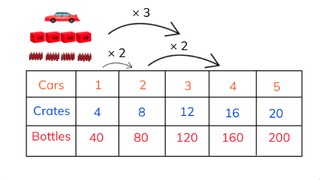Ratio tables with 3 rows

# Ratio tables with 3 rows

Students learn to solve ratio tables with three rows.

No account needed.8,000 schools use Gynzy92,000 teachers use Gynzy1,600,000 students use Gynzy

## General

Learn about ratio tables with three rows and how to solve for blanks in ratio tables with three rows.

## Standards

CCSS.Math.Content.6.RPA.3.A

## Learning objective

Students will be able to create and solve ratio tables with three rows.

## Introduction

Practice multiplying and dividing using the images given. Remind students that multiplication and division problems are related, that they reflect each other.

## Instruction

Show the image with the car and 4 crates with 10 bottles each. Then show students how you use these numbers to create a table with three rows. Then explain the steps of calculating the ratios. Next, explain to students that, when you don't know the contents of 1 square, that you can solve the ratio using a division problem. Have students try this individually or in pairs. The first exercise has thought bubbles to help support their thinking, the second exercise does not. In this way students build their confidence with ratio tables with three rows.

Check that students are able to create and solve ratio tables with three rows by asking the following questions:
- Can you name a situation in which a ratio table with three rows would be used?
- What kind of problem do you make when you want to know how many are in a in a group when you want to make 3 groups?

## Quiz

Students are given a set of 10 questions. They all have ratio tables with three rows and students must either complete the tables or solve for gaps in the tables.

## Closing

Have students form pairs. They are given numbers and should trade to complete the ratio tables. Then students are asked to create a ratio table for their partner, and ask them to complete the ratio table.

## Teaching tips

Students who have difficulty with this learning goal can be supported by making the examples very concrete and recognizable, like a bike, with two wheels, and 8 spokes per wheel, or of a farm, with 5 chickens and 20 eggs (or a zoo).

## Instruction materials

Optional: Tactile manipulatives to make the ratio tables concrete for students (so bike, chicken coop or zoo)

### The online teaching platform for interactive whiteboards and displays in schools

• Save time building lessons

• Manage the classroom more efficiently

• Increase student engagement